Courses

# Minimization of DFA Computer Science Engineering (CSE) Notes | EduRev

## Computer Science Engineering (CSE) : Minimization of DFA Computer Science Engineering (CSE) Notes | EduRev

The document Minimization of DFA Computer Science Engineering (CSE) Notes | EduRev is a part of the Computer Science Engineering (CSE) Course Theory of Computation.
All you need of Computer Science Engineering (CSE) at this link: Computer Science Engineering (CSE)

Part IV. Minimization of DFA

For a given language, many DFAs may exist that accept it. The DFA we produce from an NFA may contain many dead states, inaccessible states and indistinguishable states. all these unnecessary states can be eliminated from a DFA through a process called minimization. For practical applications, it is desirable that number of states in the DFA is minimum.

The algorithm for minimising a DFA is as follows:
Step 1: Eliminate any state that cannot be reached from the start state.
Step 2: Partition the remaining states into blocks so that all states in the same block are equivalent, and no pair of
states from different blocks are equivalent.

The process is demonstrated using an example:
Example 1:
Minimise the following DFA,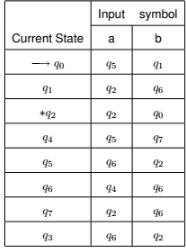Step 1: Eliminate any state that cannot be reached from the start state.
This is done by enumerating all the simple paths in the graph of the DFA beginning from the start state. Any state that is not part of some path is unreachable. In the above, the state q3 cannot be reached. So remove the corresponding to q3 from the transition table.
Now the new transition table is,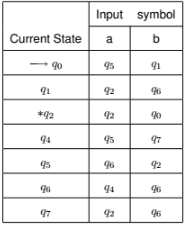Step 2:
Divide the rows of the transition table into 2 sets as,
1. One set containing only those rows which starts from non-final states.
Set 1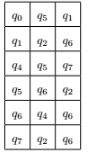2. Another set containing those rows which start from final states.
Set 2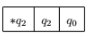Step 3a. Consider Set 1.
Find out the similar rows: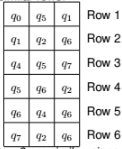Row 2 and Row 6 are similar, since q1 and q7 transit to same states on inputs a and b. So remove one of them (for instance, q7) and replace q7 with q1 in the rest.
We get,
Set 1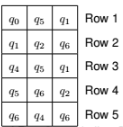Now Row 1 and Row 3 are similar. So remove one of them (for instance, q4) and replace q4 with q0 in the rest.
We get,
Set 1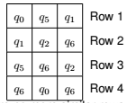Now there are no more similar rows.

Step 3b.
Consider Set 2,
Set 2Do the same process for Set 2.
But it contains only one row. It is already minimized.

Step 4:
Combine Set 1 and Set 2
We get,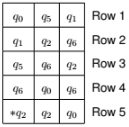The DFA corresponding to this is,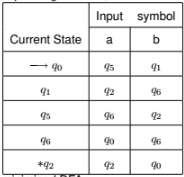Now this is a minimised DFA.
Transition diagram is,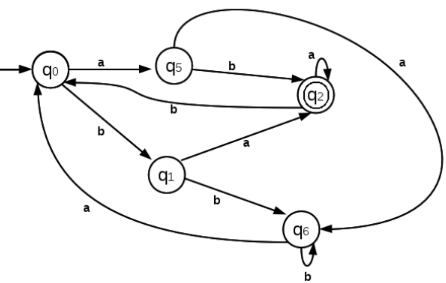Example 2:
Minimise the following DFA,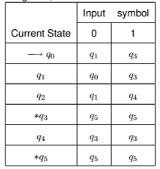Step 1: Eliminate any state that cannot be reached from the start state.
This is done by enumerating all the simple paths in the graph of the DFA beginning from the start state. Any state that is not part of some path is unreachable. In the above, the states q2 and q4 cannot be reached. So remove the rows corresponding to q2 and q4 from the transition
table.
We get,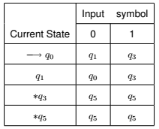Step 2: Divide the rows of the transition table into 2 sets as,
1. One set containing only those rows which starts from non-final states.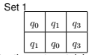2. Another set containing those rows which start from final states.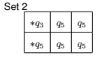Step 3a. Consider Set 1.
Find out the similar rows:
Set 1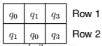There are no similar rows.

Step 3b. Consider Set 2.
Find out the similar rows: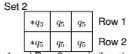Row 1 and Row 2 are similar, since q3 and q5 transit to same states on inputs 0 and 1.
So remove one of them (for instance, q5) and replace q5 with q3 in the rest.
We get,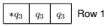Step 4:
Combine Set 1 and Set 2
We get,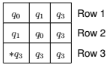The DFA corresponding to this is,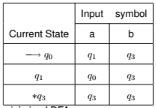Now this is a minimised DFA.
Transition diagram is,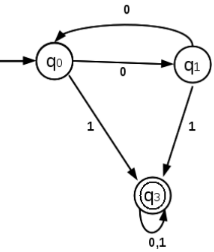Exercise:
1. Minimise the following DFA represnted as transition table,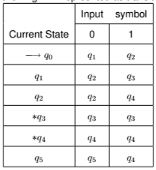2. Minimise the following DFA represnted as transition table,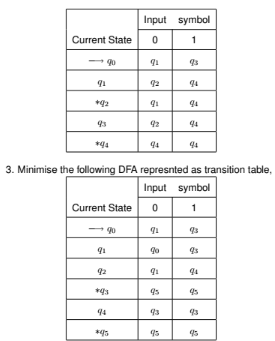4. Minimise the following DFA represnted as transition table,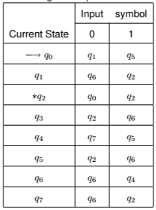5. Minimise the following DFA represnted as transition table,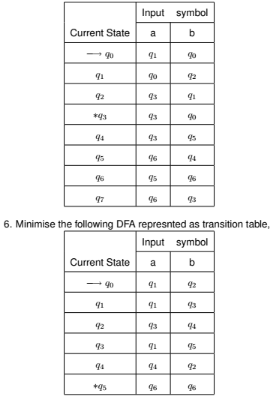7. Minimise the following DFA represnted as transition table,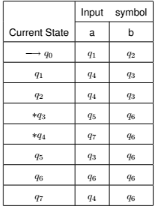Offer running on EduRev: Apply code STAYHOME200 to get INR 200 off on our premium plan EduRev Infinity!

## Theory of Computation

18 videos|43 docs|39 tests

,

,

,

,

,

,

,

,

,

,

,

,

,

,

,

,

,

,

,

,

,

;